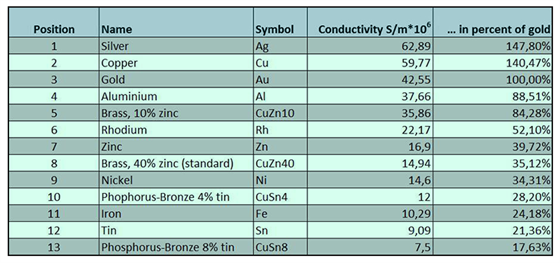# Specific Conductivity And Molar ConductivityThis topic explains about the specific conductivity and molar conductivity. The conductance of material is the property of materials due to which a material allows the flow of ions through itself and thus conducts electricity. It is generally defined as the reciprocal of resistance of that material. SI unit of conductance is S (Siemens). Specific conductivity (better known as conductivity) is the measure of the ability of that material to conduct electricity. It is represented by the symbol “К”. Hence, by definition,

G = $\frac 1R$

R= ρ$\frac 1A$

К= $\frac 1ρ$

G =К $\frac Al$

Where,

К = conductivity,

ρ = resistivity of the material

G= conductance

R= resistance

l = length

A= area of cross-section

The conductance of a material depends on the nature of the material, no. of valence electrons for a material and temperature. Metals are good conductors of electricity due to their valence electrons. We observe that the conductance of materials decreases with increase in temperature.

Water in its pure state is known to have very low conductivity due to the presence of hydroxyl ions. The presence of electrolytes further enhances the conductivity as they furnish their ions in the solution. The conductance of electricity by ions present in the solution is called electrolytic or ionic conductance. Specific conductivity or conductivity of an electrolytic solution at any given concentration is the conductance of one unit volume of solution kept between two platinum electrodes with the unit area of cross-section and at a distance of unit length. The conductivity of electrolytic solutions depends on:

1. The nature and the concentration of the electrolyte added
2. The size of the ions produced and their salvation.
3. Solvent nature and viscosity.
4. Temperature.

Due to charge, concentration and size of the ions in which electrolytes dissociate or ease with which the ions move under a potential gradient, the conductivity of solutions of different electrolytes differs with the same solvent and at a given temperature. Hence, we define a more common term molar conductivity for an electrolyte solution. The molar conductivity of a solution at a given concentration is the conductance of the volume of solution containing one mole of electrolyte kept between two electrodes with the unit area of cross-section and distance of unit length. In general terms, it is defined as the ratio of specific conductivity and the concentration of the electrolyte. The symbol Ʌm denotes it.

Ʌm= $\frac Kc$

Where,

К = specific conductivity

c= concentration of electrolyte.

For detail discussion on specific and molar conductivity, download BYJU’S – The Learning App.